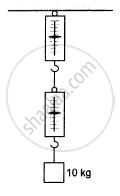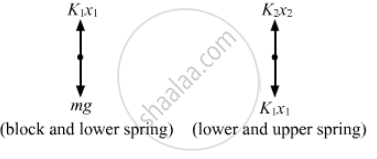# A Block of Mass 10 Kg is Suspended from Two Light Spring Balances, as Shown in the Figure. - Physics

MCQ

A block of mass 10 kg is suspended from two light spring balances, as shown in the following figure.#### Options

• Both the scales will read 10 kg.

• Both the scales will read 5 kg.

• The upper scale will read 10 kg and the lower zero.

• The readings may be anything but their sums will be 10 kg.

#### Solution

Both the scales will read 10 kg.From the free-body diagram
K1x1 = mg = 10 x 9.8 = 98 N
K2x2 = K1x1
So,  K1x1 = K2x2 = 98 N

Therefore, both the spring balances will read the same mass, i.e. 10 kg.

Concept: Newton's First Law of Motion
Is there an error in this question or solution?

#### APPEARS IN

HC Verma Class 11, Class 12 Concepts of Physics Vol. 1
Chapter 5 Newton's Laws of Motion
MCQ | Q 4 | Page 77